## Evaluating Limits

### Methods of evaluation of limits

We shall divide the problems of evaluation of limits in five categories.

### (1) Algebraic limits:

Let f(x) be an algebraic function and ‘a’ be a real number. Thenis known as an algebraic limit.

1. Direct substitution method: If by direct substitution of the point in the given expression we get a finite number, then the number obtained is the limit of the given expression.
2. Factorisation method: In this method, numerator and denominator are factorised. The common factors are cancelled and the rest outputs the results.
3. Rationalisation method: Rationalisation is followed when we have fractional powers (like $$\frac { 1 }{ 2 } ,\frac { 1 }{ 3 }$$ etc.) on expressions in numerator or denominator or in both. After rationalisation the terms are factorised which on cancellation gives the result.
4. Based on the form when x → ∞: In this case expression should be expressed as a function 1/x and then after removing indeterminate form, (if it is there) replace 1/x by 0.

### (2) Trigonometric limits:

To evaluate trigonometric limit the following results are very important.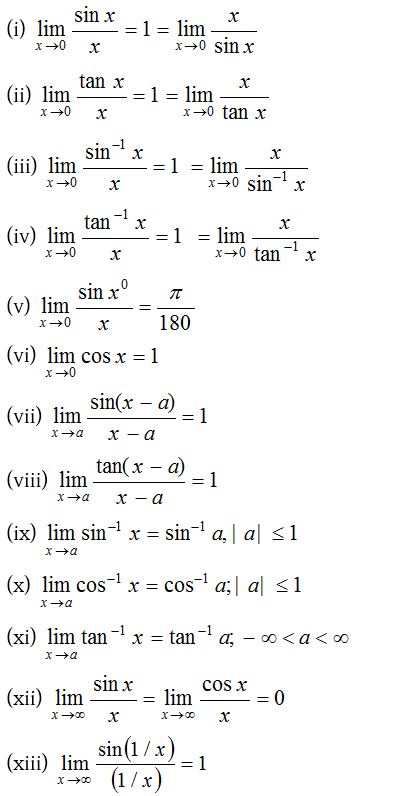### (3) Logarithmic limits:

To evaluate the logarithmic limits we use following formulae: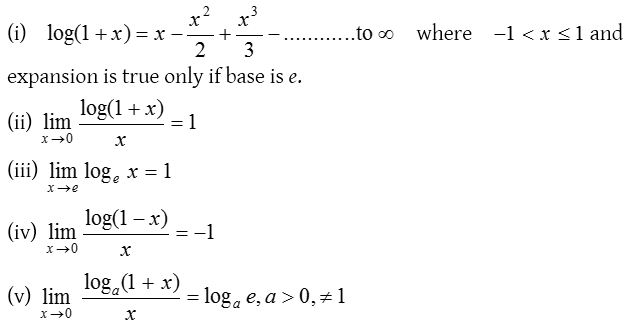### (4) Exponential limits:

(i) Based on series expansion: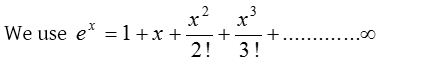To evaluate the exponential limits we use the following results:(ii) Based on the form 1: To evaluate the exponential form 1 we use the following results.### (5) L-Hospital’s rule:

If f(x) and g(x) be two functions of x such thatSometimes it may be necessary to repeat this process a number of times till our goal of evaluating limit is achieved.

### Evaluating Limits Problems with Solutions

1.Solution:2.Solution: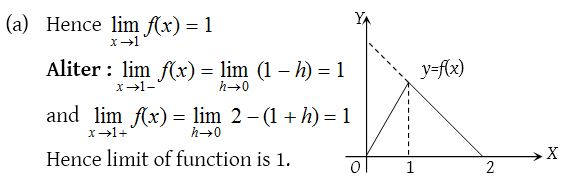3.Solution: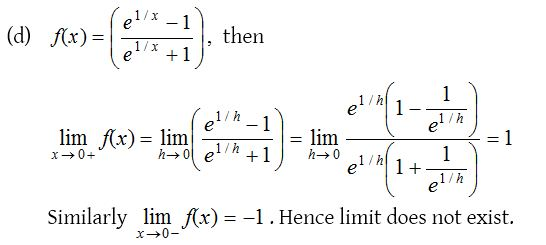4.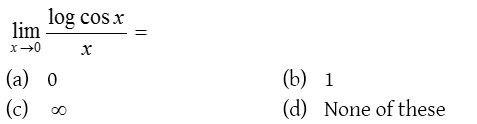Solution: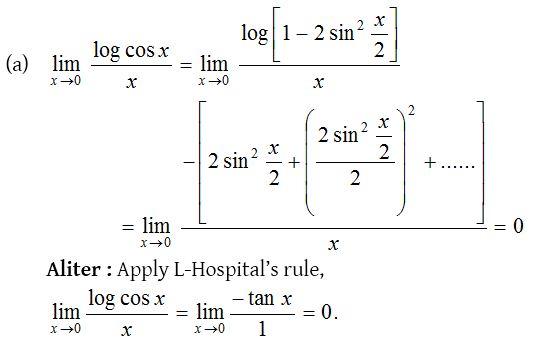5.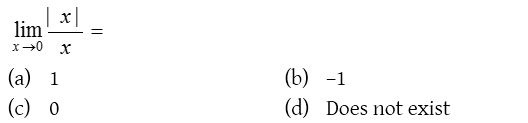Solution:6.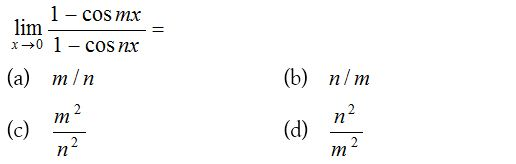Solution: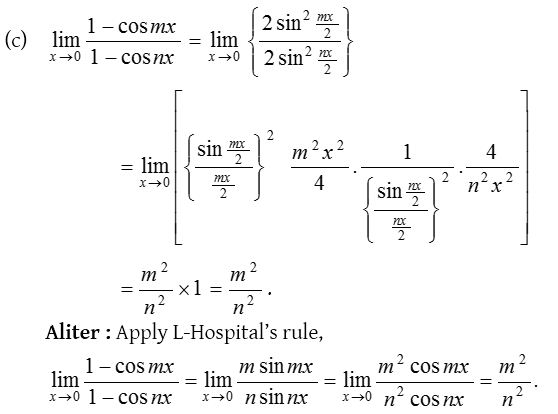7.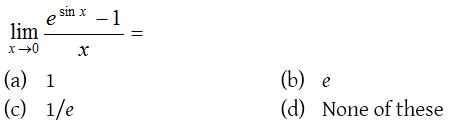Solution: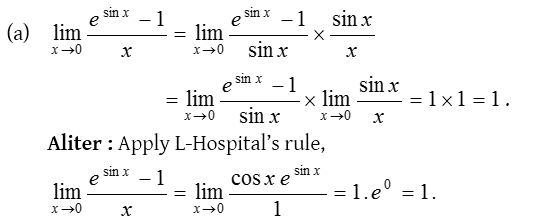8.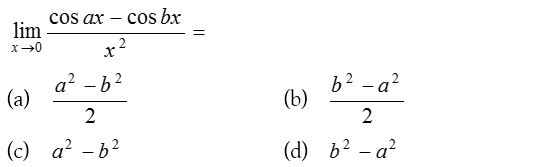Solution: